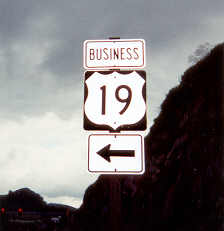# 19

This number is a prime.

Just showing those entries submitted by 'Gaydos': (Click here to show all)There are 19 Sophie Germain primes whose sums of reciprocals of digits equal one. The smallest is 86843 and the largest is 98689889. [Gaydos]The binary representation of 10^19 contains 19 ones. [Gaydos]There are 19 brilliant numbers with digits in strictly descending order. [Gaydos]The sum of the four 3-letter integers (one+two+six+ten). [Gaydos]19^19 is in a prime gap of length 19. [Gaydos]

(There is one curio for this number that has not yet been approved by an editor.)